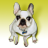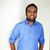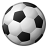Announcements
You can succeed best and quickest by helping others to succeed. Join the conversation.
cancel
Showing results for
Did you mean:Creator

## Set Analysis - Exclude some values but keep nulls

Hi,

My set analysis is like this:

= Sum(

{<

Type -= {'ABC','XYZ','PQR'},

ACT_NM = ,

Date = ,

Month = {'\$(vMaxMonth'}

>}

Amount)

Some Account has Null values (-) in Type field but they have the Amount values. When i use this set analysis i dont get the account amount value that has null(-) value in Type field.

How do i get the Amount value for the Null values in the straight chart?

Any help is highly appreciated.

Straight chart:

Account ID       Type             Amount

1001                      ABC              100

1002                     XYX                200

1003                    PQR               150

1004                      -                     180

Thanks,

BC

1 Solution

Accepted SolutionsCreator III

These things  will work

1.Sum(

{<

ACT_NM = ,

Date = ,

Month = {'\$(vMaxMonth'}

>}

Amount)-

Sum(

{<

Type = {'ABC','XYZ','PQR'},

ACT_NM = ,

Date = ,

Month = {'\$(vMaxMonth'}

>}

Amount)

2.

Sum(

{<

Type =- {'ABC','XYZ','PQR'},

ACT_NM = ,

Date = ,

Month = {'\$(vMaxMonth'}

>}

Amount)+

Sum(

{<

ACT_NM = ,

Date = ,

Month = {'\$(vMaxMonth'}

>}

if(isnull(Type),Amount))

3. assign Nullasvalue in the script

5 RepliesCreator III
Instead of SUM try COUNT and for ACT_NM = , Date = , try
ACT_NM = '', Date = '', or ACT_NM ={''} , Date ={''} ,...Creator III

These things  will work

1.Sum(

{<

ACT_NM = ,

Date = ,

Month = {'\$(vMaxMonth'}

>}

Amount)-

Sum(

{<

Type = {'ABC','XYZ','PQR'},

ACT_NM = ,

Date = ,

Month = {'\$(vMaxMonth'}

>}

Amount)

2.

Sum(

{<

Type =- {'ABC','XYZ','PQR'},

ACT_NM = ,

Date = ,

Month = {'\$(vMaxMonth'}

>}

Amount)+

Sum(

{<

ACT_NM = ,

Date = ,

Month = {'\$(vMaxMonth'}

>}

if(isnull(Type),Amount))

3. assign Nullasvalue in the scriptCreator III

1.Replace the null values with 'Null' text in the backend in the following way

if (isnull(Type), 'Null', Type) as Type

2.Include 'Null' in the set analysis

Hope this helps. Please post some sample data if this doesnt resolve the issue.

Regards,

ArpitCreator
Author

Hi Asinha1991 ,

Yes, your solution is giving me the correct numbers.

Thanks a lot.

BCCreator III
yw 🙂Community Browser